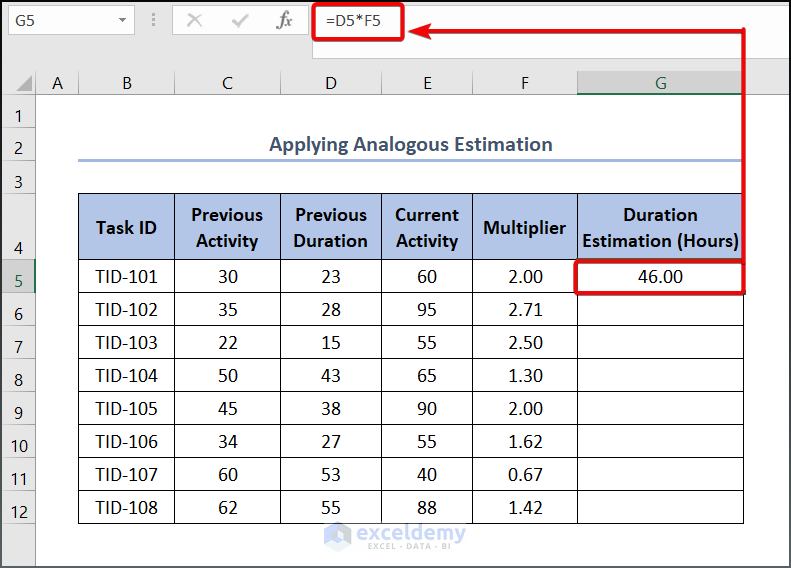# How to Make an Effort Estimation Sheet in Excel (4 Easy Methods)

Get FREE Advanced Excel Exercises with Solutions!

Excel plays a vital role in business analysis as it opens a wide range of opportunities to store data and analyze them accordingly. Apart from that, it is a useful, cost-saving, and tractable tool, thanks to numerous options and features embedded within it. Today we will talk about how to prepare an effort estimation sheet in Excel. So, stick with us to have a brief discussion of it.

## What Is Effort Estimation?

Effort Estimation is a conventional process to forecast the required effort to complete a project. This is traditionally measured by the hours.

The key definitions we must understand before delving into our today’s topic such as:

Parametric Estimation:  Parametric Estimation is a project estimation technique for cost estimation, duration of the project, and involvement effort in the task. It is one of the four primary techniques used by project managers when estimating a project which involves a set of computations, statistics, or algorithms to describe the project.

Analogous Estimation: When there is little data available for a project, it is a tiresome job to make an accurate estimation. But utilizing an analogous technique helps you to estimate your effort. You can estimate outcomes and make plans accordingly by comparing current projects to past projects.

Triangular or Three-point Distribution: This is also another technique when you have little access to the relevant dataset. Not to mention creating a normal distribution graph when you have a wide range of data, you can estimate your effort based on the three-point technique.

PERT BETA Distribution: The PERT approach enables the three-point estimate to be transformed into a normally distributed shape, whereas the triangular distribution simply takes into account the three different points. It can therefore be used to calculate the probabilities of different expected duration ranges.

## 4 Methods to Prepare Effort Estimation Sheet in Excel

Let’s assume we have a dataset, namely “Effort Estimation Sheet for Dunder Mifflin Limited”. You can use any dataset suitable for you.Here, we have used the Microsoft Excel 365 version; you may use any other version according to your convenience.

### 1. Using Parametric Estimation

Unlike Analogous Estimation here in Parametric Estimation, we will not rely on historical data rather than will use some variables such as percent of availability, performance factor, etc. In the case of the performance factor, the average value of it is equal to 1.0. More skilled the lower the factor e.g. 0.8, less skilled higher e.g. 1.2.

📌 Steps:

• To begin with this method, enter the following formula in cell G5.

`=C5/(D5*E5*F5)`### 2. Applying Analogous Estimation

Analogous Estimation is made based on historical data like the same work in different working conditions or scales. One disadvantage of this method is its inaccuracy, thus a decision-maker may need to verify the calculation with other models to get a reasonable solution.

📌 Steps:

• Write the following formula in cell F5.

`=E5/C5`• To get the other value, drag the Fill Handle tool from F5 to F12.• Now to calculate the duration, enter the following formula in cell G5.

`=D5*F5`

###• Lower the cursor to the C12 cell to use the AutoFill tool.### 3. Utilizing Three-Point Estimation

Triangular Estimation aka three-point estimation is a common technique used by professionals when there is limited data available in the effort estimation analysis.

The basic formula can be described as follows:

E = (O + B + P ) / 3

where E is Estimate;
O = Optimistic Duration (which is the potential workload that benefits to materialize)
P = Pessimistic Duration ( which represents the potential outcome if the negative factors occur)
M = Best Guess ( This represents the typical amount of time the task would require if performed 100 times by the team member.)

📌 Steps:

• Write the following formula in cell F5.

`=``(C5+D5+E5)/3`• Drag the Fill Handle tool to get the other values.### 4. Incorporating PERT BETA Distribution

The PERT BETA Distribution is a weighted average where the most likely event gets the maximum weight. Unlike Triangular Estimation, it provides better estimation when you have limited data resources.

Thus similar to the previous method the formula of PERT BETA Distribution is as follows

E = (O + 4B + P ) / 6

📌 Steps:

• To begin with this method, enter the following formula in cell F5.

`=(C5+4*D5+E5)/6`• To get the other value, drag the Fill Handle tool from F5 to F12.## Practice Section

We have provided a Practice section on the right side of each sheet so you can practice yourself. Please make sure to do it yourself.## Conclusion

In this article, we have discussed how to prepare an effort estimation sheet in Excel. As you have already understood, there are a number of ways to do this task. So before going through a specific method, consider all the possibilities before making any final decision. Further, If you have any queries, feel free to comment below and we will get back to you soon. Also, you may follow our website, ExcelDemy, a one-stop Excel solution provider to explore more.

## Related ArticlesMiran is a highly motivated individual with a strong educational background in engineering. He is interested in technology and passionate about creating engaging and informative content. After graduation, Miran decided to pursue a career in content development and has been working in the field for some time. He is eager to continue learning and growing as a professional.

We will be happy to hear your thoughtsAdvanced Excel Exercises with Solutions PDF